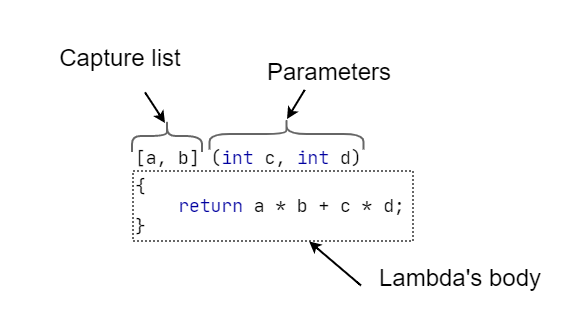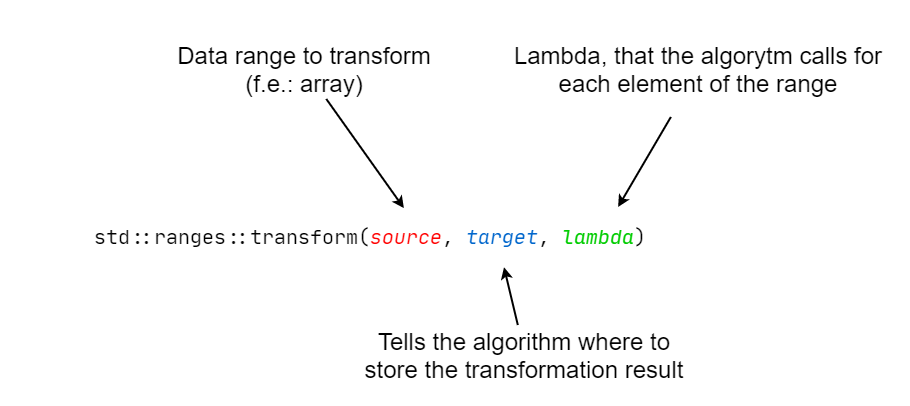# Lambda expressions

Lambda expression, more often just called "lambdas", are a convenient way to write a snippet of code in a form of an object, so that it can be sent as a function argument and reused later. Lambdas are mostly used for:

• creating named objects inside functions, that can be later reused just like functions, without polutting the global namespace.
• creating "anonymous" snippets of code that can be sent to other functions (e.g. standard library algorithms).

We recommend to look at Simple examples and Practical usage for some examples.

Anonymous functions, functors, function objects

Lambdas are often called anonymous functions, functors or function objects. None of these names are correct, although can be used when talking about lambdas. Indeed, lambdas create an invisible object, although they are only expressions themselves. Because of the way lambdas work (creating a magic, invisible object, of some magic, not-known type), to assign them to an object, we need to use the keyword `auto`, or make use of the standard library type - `std::function` (we will learn about it further in the course).

## The syntax​A lambda must have a body, in which we will write our code and a capture list (that can be empty). The parameter list is optional, although very often used. We can add various other things to the lambda expression, like attributes, explicit return type, etc., although they are neither mandatory nor often used, so we will talk about them further in the course.

#### Capture list​

As we know from the lesson about functions, local variables (e.g. from the `main` function) are not known in the body of any other function. The same thing applies to the lambda expressions. Local variables from a function are not visible inside a lambda expression, that's why they need to be captured in the capture list.

A lambda with a capture list
``int five = 5;auto get7 = [five] () { return five + 2; };std::cout << get7();``
Result (console)
``7``
note

In case of a lambda expression with an empty parameter list, the parentheses can be ommited.

``int five = 5;auto get7 = [five] { return five + 2; };std::cout << get7();``
Mutating the captured variables

The variables captured in the capture list cannot be changed for now. There's a way to do that, but we will talk about it in the second course lesson.

#### Parameter list​

The parameter list in the lambda expression works just like the one we know from functions. It allows us to declare with what parameters our lambda should be called, and then pass arguments to it.

A lambda with a parameter list.
``auto multplyBy7 = [] (int a) { return a * 7; }; // a lambda with a parameter of type intstd::cout << multplyBy7(5); // the lambda called with an argument 5``
Result (console)
``35``

#### The lambda body​

Just a conventional code block, that we already know. Here we declare variables, make operations on objects, etc. We can use the `return` statement inside the lambda body.

### Simple examples​

#### A comparision of a lambda and a function returning 5 with every call​

Lambda
``#include <iostream>int main(){    auto five = [] { return 5; };    std::cout << five();}``
Function
``#include <iostream>int five() {    return 5;}int main(){    std::cout << five();}``

#### A lambda returning the square of its argument​

Lambda expression with a parameter
``auto square = [](int x) { return x*x; };std::cout << square(5);``
Result (console)
``25``

#### Lambda used for code reusage​

Lambda as a function in function
``void print3Hellos(std::string name) {    auto print_hello = [name](std::string hello) {        std::cout << hello << ", " << name << "!\n";    }    print_hello("Hello");    print_hello("Welcome");    print_hello("Hi");}// ...print3Hellos("Mark");``
Result (console)
``Hello, Mark!Welcome, Mark!Hi, Mark!``

## Common mistakes​

### Trying to use a non-captured variable​

Trying to use a non-captured variable
``int main(){    int A = 5;        // ❌ Variable A is not known inside addToA ❌     // auto addToA = [] (int b) { return A + b; };    // ✅ Proper lambda declaration ✅    auto addToA = [A] (int b) { return A + b; };    std::cout << addToA(5) << "\n";}``

### Trying to modify a captured variable​

Trying to modify a captured variable
``int main(){    int A = 5;        // ❌ We can't modify the variable A ❌    // auto addToA = [A] (int b) { A += b; };    // ✅ For now, we can make use of the fact that we can return values.    // You will learn how to modify captured variables later in the course. ✅    auto addToA = [A] (int b) { return A + b; };    std::cout << addToA(5) << "\n";}``

## Practical usage​

C++ version

We suggest to use the newest C++ version (properly called a standard) - C++20, because it provides a lot of convenient features. If you can't use C++20 for some reason, we also provide examples that work on older versions.

### Using a lambda with the transform algorithm​

To use this algorithm, you have to include the `algorithm` header.

``#include <algorithm>``

#### The goal​

In the example, we will create a vector of numbers and square every each of it with the use of the transform algorithm.

#### The way to go​

The `transform` algorithm, can be passed a function, function object, or a lambda. Since it's a lesson about lambdas, we will make use of them. Our lambda will take one parameter of type `int` and will return a value of the same type.The `ranges` namespace

Since C++20, we can use the more convenient version of the algorithm that's located in the `ranges` namespace, that's why we have to write `std::ranges::transform`, instead of `std::transform`.

#### 1. The source​

The first argument is the source of the data - in our case it's a vector of ints.

``std::vector<int> data = {1, 2, 3, 4, 5};std::ranges::transform(data, [...]);``

#### 2. The destination​

The second argument is the beginning to a container that we want to save the data to. The other container has to have the same or bigger size, as the source container. We can use the iterator from our `data` vector, or from some other one.

``std::vector<int> result;result.resize(data.size());std::ranges::transform(data, result.begin(), [...]);    // Also correct ✅std::ranges::transform(data, data.begin(), [...]);``

#### 3. The lambda​

The most important part of the algorithm, the third argument. We send a lambda that:

• Takes one parameter of the same type as the source container (`int` in this case)
• Returns a value of the same type as the destination container (also `int` in this case)
``auto square = [](int a) { return a * a; };std::ranges::transform(data, result.begin(), square);// We can also pass the lambda directly, without first saving it into an object:std::ranges::transform(data, result.begin(), [](int a) { return a * a; });``

#### 4. The whole example​

Squaring a vector
``#include <iostream>#include <vector>#include <algorithm>int main(){    std::vector<int> data = {1, 2, 3, 4, 5};    std::cout << "Before using the algorithm:\n";    for(auto elem : data)    {        std::cout << elem << " ";    }    std::cout << "\n\n";    auto square = [](int a) { return a * a; };    std::ranges::transform(data, data.begin(), square);    std::cout << "After using the algorithm:\n";    for(auto elem : data)    {        std::cout << elem << " ";    }}``
Result (console)
``Before using the algorithm:1 2 3 4 5After using the algorithm:1 4 9 16 25``